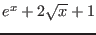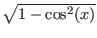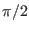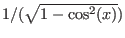Next: Continuation for one-dimensional system Up: ALIAS-Maple Previous: Optimization

• La page de J-P. Merlet
• La page Présentation de HEPHAISTOS
• La page "Présentation" de l'INRIA

Subsections

# Integration

ALIAS provides various procedures for the certified calculation of definite integrals. These procedures will return a range that is guaranteed to include the real value of the integral. The width of the range will be lower than the global variable ALIAS/fepsilon. In the current implementation only finite bounds may be used.

Some of the following procedures involve the use of the derivatives and you should be careful when the interval evaluation of these derivative cannot be performed. For example when consideringyou should not 0 in the integration domain. In that case you should integrate around 0 by using the Integrate problem while using another procedure for the remaining part of the domain.

# Integral in one variable

The basic integration procedure is Integrate(Func , Init) where Func is the integrand and Init the range for the integration. For example


Integrate(1/x,[1,2]);

returns the range [.69264842756679057, .69364643155883843] if ALIAS/fepsilon is set to 1e-3. This procedure should not be used to determine accurate range.

For accurate computation for function that are at least twice differentiable you may use IntegrateTrapeze or IntegrateRectangle which have the same syntax than Integrate. You may also use IntegrateTaylor that use a Taylor expansion of order N of the function. The syntax is


IntegrateTaylor(Func,Init,N)


All these procedures will return UNABLE if the integral cannot be computed with the desired accuracy or FAILED if the number of boxes exceed the global variable ALIAS/maxbox. As the default value of this variable is 20 000 (i.e. relatively small) it may be necessary to set the variable to a larger number before calculating the integral.

Note that the derivatives of the function and the function itself may involve expression that cannot be interval evaluated everywhere. Hence it is of good policy to check this point by using the Problem_Expression package and that may require to set some parameters of this package (see section 2.1.5).

For example assume that we have to integrate the functionin the range 0,. The derivatives of this expression involves the expression ofwhich is not defined in 0. If we use IntegrateTaylor of order 2 we need to ensure that the function and the derivatives up to the third one can be interval evaluated.


eq:=sqrt(1-cos(x)^2):
ALIAS/close_to_zero:=1e-20:
ALIAS/low_value_expr_violated:=1:
ALIAS/high_value_expr_violated:=1:
Verify_Problem_Expression([eq,diff(eq,x\$1),diff(eq,x\$2),diff(eq,x\\$3)],[x]):
IntegrateTaylor(eq,[0,evalf(Pi)/2.],2);

The setting of the parameters allows to calculate the integral.

# Integral in several variables

The basic multiple integral procedure of ALIAS is


IntegrateMultiRectangle(Func,Vars,Init)

that may be used as in this example:

IntegrateMultiRectangle(x*sin(y),[x,y],[[0,1],[0,1]]);

This procedure requires that the function is at least twice differentiable.

A more sophisticated procedure is based on Taylor expansion. Its syntax is


IntegrateMultiTaylor(Func,Vars,Init,N,Type)

where N is the order used for the Taylor expansion. If N is large (i.e. say larger than 6) the remainder of the Taylor expansion may be a very large expression, that may be difficult to compile. Type, which should either "explicit" or "autodiff", may be used to deal with this problem. If it is set to "explicit" the remainder will be calculated exactly and be compiled as it is. If Type is set to "autodiff" the procedure will try to use forward automatic differentiation and elementary components identification (see the procedures Decompose_Diff and Auto_Diff) to reduce the compilation time. Still you cannot expect to be able to use very large value for N if the integrand is complicated.Next: Continuation for one-dimensional system Up: ALIAS-Maple Previous: Optimization